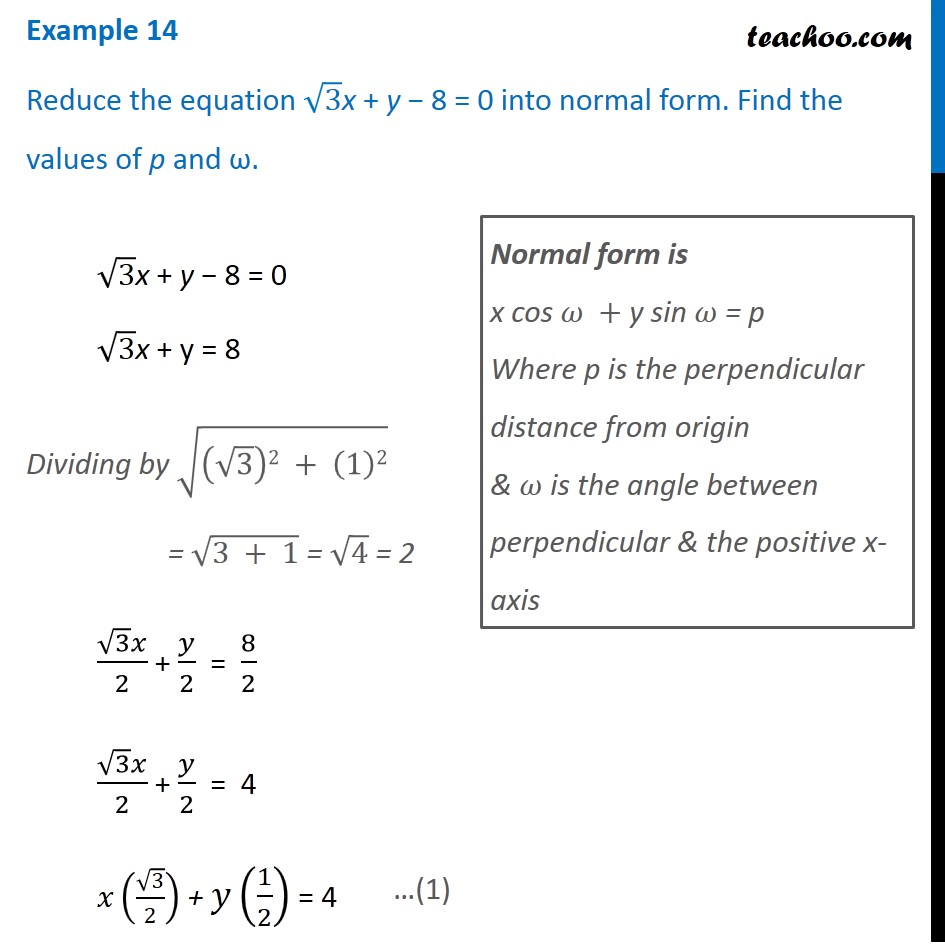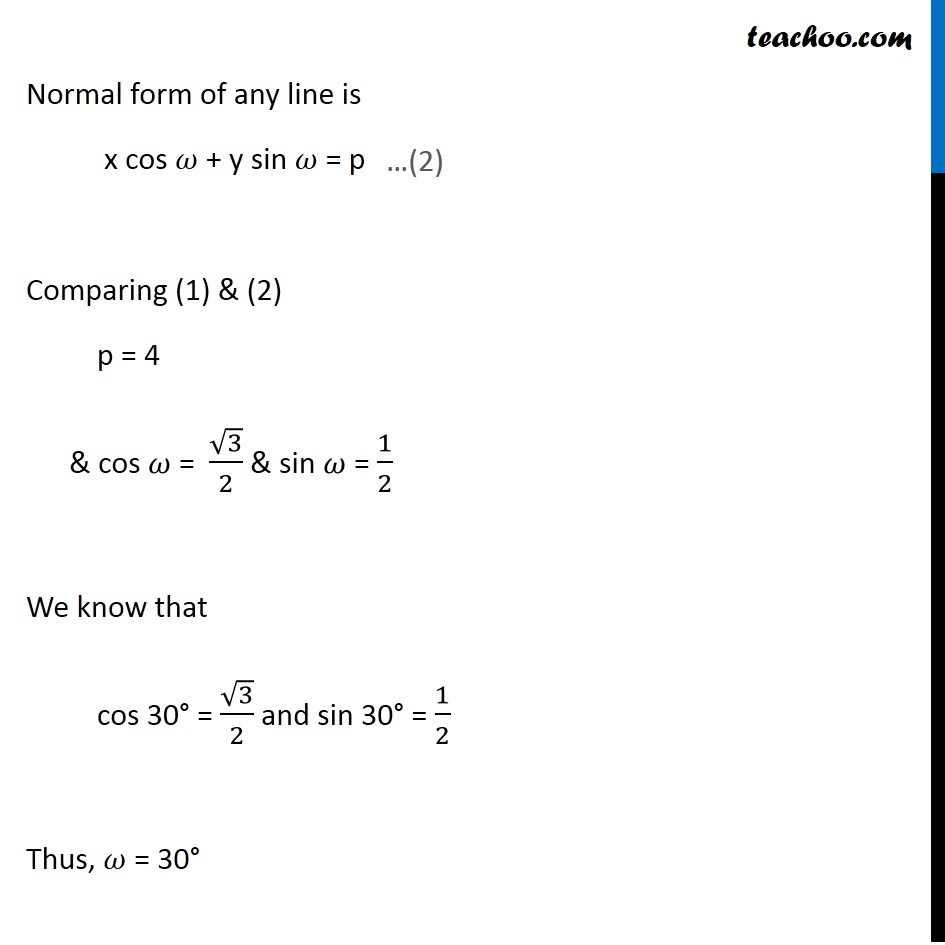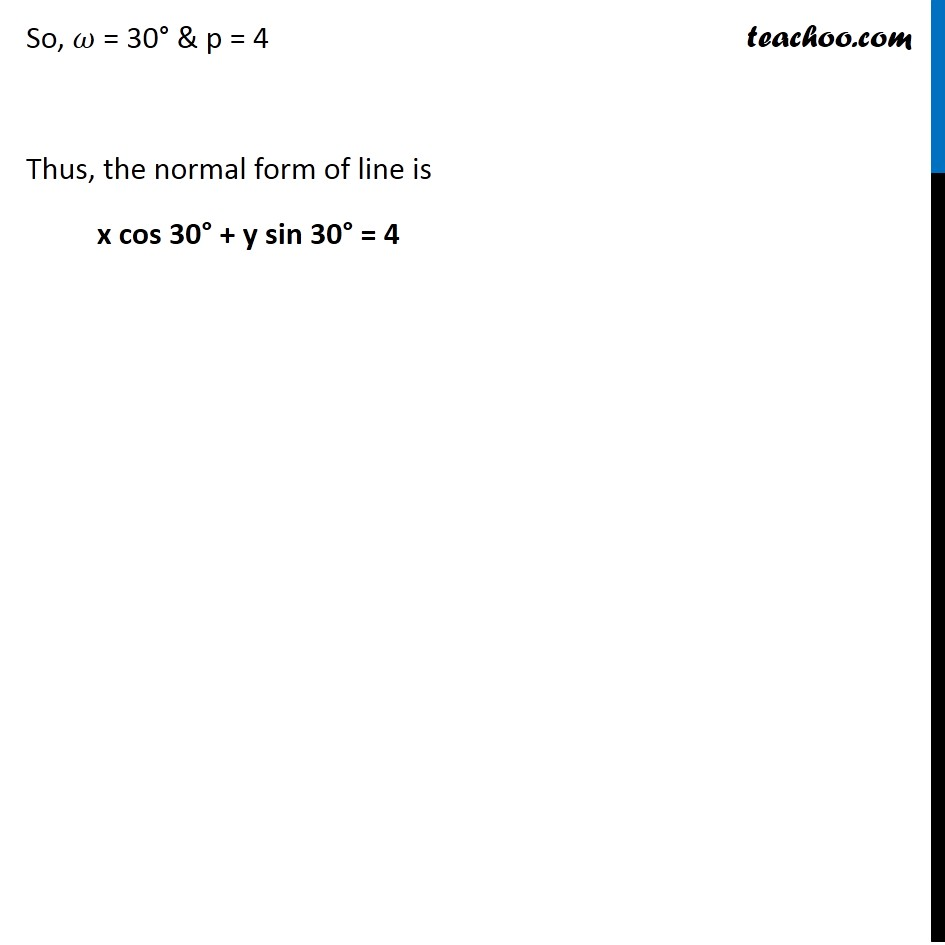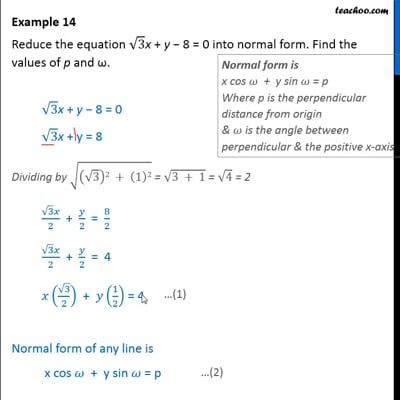Examples

Chapter 10 Class 11 Straight Lines
Serial order wiseThis video is only available for Teachoo black users

Solve all your doubts with Teachoo Black (new monthly pack available now!)

### Transcript

Example 14 Reduce the equation √3x + y − 8 = 0 into normal form. Find the values of p and ω. √3x + y − 8 = 0 " " √3 "x + y" = 8 Dividing by √((√3)2 + (1)2) = √(3 + 1) = √4 = 2 (√3 𝑥)/2 + 𝑦/2 = 8/2 (√3 𝑥)/2 + 𝑦/2 = 4 𝑥(√3/2) + 𝑦(1/2) = 4 Normal form is x cos 𝜔 + y sin 𝜔 = p Where p is the perpendicular distance from origin & 𝜔 is the angle between perpendicular & the positive x-axis Normal form of any line is x cos 𝜔 + y sin 𝜔 = p Comparing (1) & (2) p = 4 & cos 𝜔 = √3/2 & sin 𝜔 = 1/2 We know that cos 30° = √3/2 and sin 30° = 1/2 Thus, 𝜔 = 30° So, 𝜔 = 30° & p = 4 Thus, the normal form of line is x cos 30° + y sin 30° = 4